Test: Division - 3

# Test: Division - 3

Test Description

## 20 Questions MCQ Test Mathematics for Class 3: NCERT | Test: Division - 3

Test: Division - 3 for Class 3 2023 is part of Mathematics for Class 3: NCERT preparation. The Test: Division - 3 questions and answers have been prepared according to the Class 3 exam syllabus.The Test: Division - 3 MCQs are made for Class 3 2023 Exam. Find important definitions, questions, notes, meanings, examples, exercises, MCQs and online tests for Test: Division - 3 below.
Solutions of Test: Division - 3 questions in English are available as part of our Mathematics for Class 3: NCERT for Class 3 & Test: Division - 3 solutions in Hindi for Mathematics for Class 3: NCERT course. Download more important topics, notes, lectures and mock test series for Class 3 Exam by signing up for free. Attempt Test: Division - 3 | 20 questions in 40 minutes | Mock test for Class 3 preparation | Free important questions MCQ to study Mathematics for Class 3: NCERT for Class 3 Exam | Download free PDF with solutions
 1 Crore+ students have signed up on EduRev. Have you?
Test: Division - 3 - Question 1

### (27 ÷ 3) × 8 = ______

Detailed Solution for Test: Division - 3 - Question 1

(27 ÷ 3) × 8 = 9 × 8 = 72

Test: Division - 3 - Question 2

### A number with "0" at one's place is divisible by:

Detailed Solution for Test: Division - 3 - Question 2

A number having 0 at one?s place is divisible by 2, 5 and 10.

Test: Division - 3 - Question 3

### How many 8's are there in 120?

Detailed Solution for Test: Division - 3 - Question 3

120 ÷ 8 = 15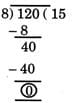Test: Division - 3 - Question 4

The least number among the following is:

Detailed Solution for Test: Division - 3 - Question 4

68 ÷ 4 = 17, 68 + 4 = 72, 68 × 4 = 272, 68 − 4 = 64 Here, 17 is the least.

Test: Division - 3 - Question 5

Anupama divided 498 by 8 and obtained a quotient of 62 and remainder 2. For this problem, which of these checks will help Anupama to find out her mistakes?

Detailed Solution for Test: Division - 3 - Question 5

In division problems, remainder should always be less than divisor.

Test: Division - 3 - Question 6

0 ∗ 18 = 0 This will be true if * is replaced by:

Detailed Solution for Test: Division - 3 - Question 6

0 × 18 = 0
0 ÷ 18 = 0

Test: Division - 3 - Question 7

If we start subtracting 4 from 19 stepwise as shown, we will be able to subtract 4 times.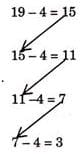If we start subtracting 4 from 169 in a similar manner, how many times will we be able to subtract?

Detailed Solution for Test: Division - 3 - Question 7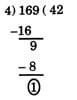Test: Division - 3 - Question 8

Ramya divides a 3-digit number by 10. What can we say about the quotient?

Detailed Solution for Test: Division - 3 - Question 8

The quotient is obtained by removing one?s digit
Ex: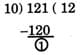By removing one?s digit of 121 we get 12.

Test: Division - 3 - Question 9

This is a seating arrangement for students in a classroom with each box showing one chair. Thy chairs which have a star mark are occupied.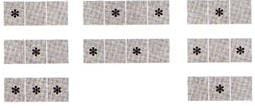Which of the following statement is true?

Detailed Solution for Test: Division - 3 - Question 9

Number of empty chairs = Number of occupied chairs = 13

Test: Division - 3 - Question 10

If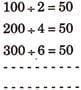then 800 ÷ 16 = _______.

Detailed Solution for Test: Division - 3 - Question 10

Observing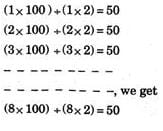Test: Division - 3 - Question 11

Raju has four plates of laddoos. He wants to put them equally in four plates. What should be the number of laddoos in each plate?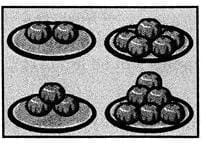Detailed Solution for Test: Division - 3 - Question 11

Total number of laddoos = 2 + 5 + 3 + 6 = 16
∴ Number of laddoos in each plate = 16/4 = 4

Test: Division - 3 - Question 12

There are 21 bananas.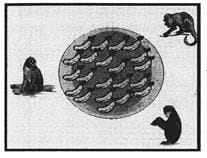What is the equal share of each monkey?

Detailed Solution for Test: Division - 3 - Question 12

Share of each monkey = 21/3 = 7

Test: Division - 3 - Question 13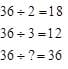Missing number is:

Detailed Solution for Test: Division - 3 - Question 13

36 + 1 = 36

Test: Division - 3 - Question 14

There are few pencils in a box. Rahul took out those pencils from the box and put them in five groups of each 4. Three pencils were left in the box. How many pencils were there in the box?

Detailed Solution for Test: Division - 3 - Question 14

Number of pencils he took out from the box = 5 × 4 = 20
Remaining pencils in the box = 3
∴ Total number of pencils in the box = 20 + 3 = 23

Test: Division - 3 - Question 15

Missing number in the following is: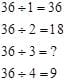Detailed Solution for Test: Division - 3 - Question 15

36 ÷ 3 = 12

Test: Division - 3 - Question 16

A shopkeeper packed 360 biscuits in 12 boxes. What is the number of biscuits in each box?

Detailed Solution for Test: Division - 3 - Question 16

Number of biscuits in each box = 360 ÷ 12 = 30

Test: Division - 3 - Question 17

If this pattern continues, then what is the next number? 80, 40, 20,  ? −−−−−−−

Detailed Solution for Test: Division - 3 - Question 17

Observe the pattern.

Test: Division - 3 - Question 18

245 candles are to be arranged equally in 15 rows. How many candles will be left?

Detailed Solution for Test: Division - 3 - Question 18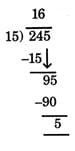5 candles will be left.

Test: Division - 3 - Question 19

If largest 3-digit number is divided by smallest 2-digit number, then:

Detailed Solution for Test: Division - 3 - Question 19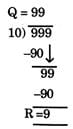Test: Division - 3 - Question 20

If 5 tens are subtracted from 300 and divided by 25, then quotient is:

Detailed Solution for Test: Division - 3 - Question 20

5 tens = 50, 3 hundreds = 300 difference = 300 - 50 = 250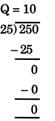## Mathematics for Class 3: NCERT

47 videos|85 docs|44 tests
Information about Test: Division - 3 Page
In this test you can find the Exam questions for Test: Division - 3 solved & explained in the simplest way possible. Besides giving Questions and answers for Test: Division - 3, EduRev gives you an ample number of Online tests for practice

## Mathematics for Class 3: NCERT

47 videos|85 docs|44 tests(Scan QR code)